# Discrete numeric functionsor numeric functions The functions whose

• Slides: 36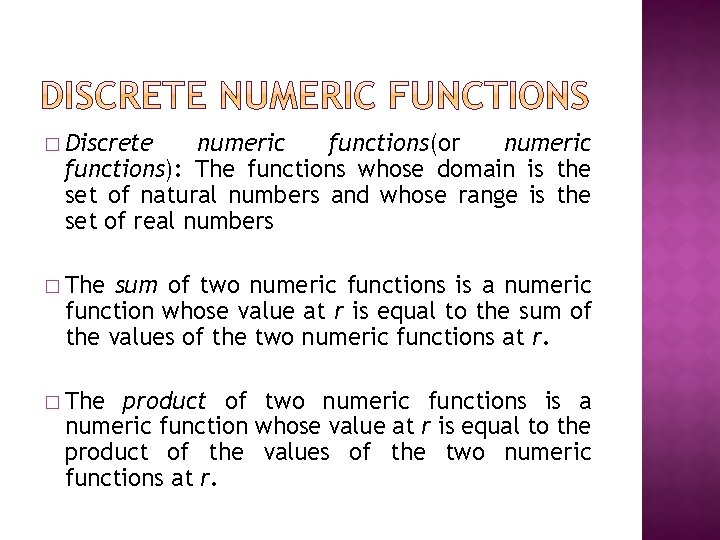� Discrete numeric functions(or numeric functions): The functions whose domain is the set of natural numbers and whose range is the set of real numbers � The sum of two numeric functions is a numeric function whose value at r is equal to the sum of the values of the two numeric functions at r. � The product of two numeric functions is a numeric function whose value at r is equal to the product of the values of the two numeric functions at r.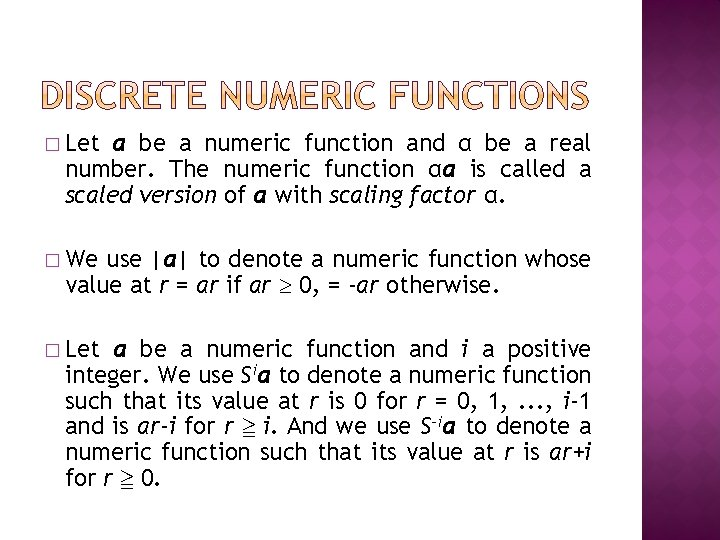� Let a be a numeric function and α be a real number. The numeric function αa is called a scaled version of a with scaling factor α. � We use |a| to denote a numeric function whose value at r = ar if ar 0, = -ar otherwise. � Let a be a numeric function and i a positive integer. We use Sia to denote a numeric function such that its value at r is 0 for r = 0, 1, . . . , i-1 and is ar-i for r ≧ i. And we use S-ia to denote a numeric function such that its value at r is ar+i for r ≧ 0.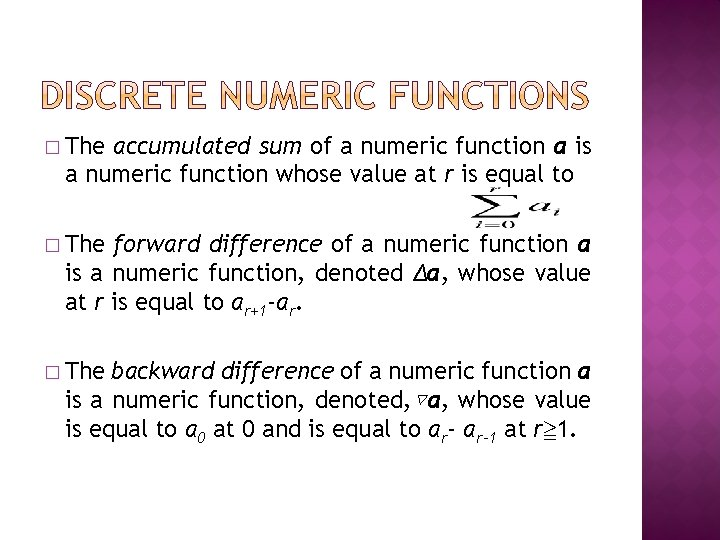� The accumulated sum of a numeric function a is a numeric function whose value at r is equal to � The forward difference of a numeric function a is a numeric function, denoted Δa, whose value at r is equal to ar+1 -ar. � The backward difference of a numeric function a is a numeric function, denoted, ▽a, whose value is equal to a 0 at 0 and is equal to ar-1 at r≧ 1.� Let a and b be two numeric functions. The convolution of a and b, denoted a*b, is a numeric function such that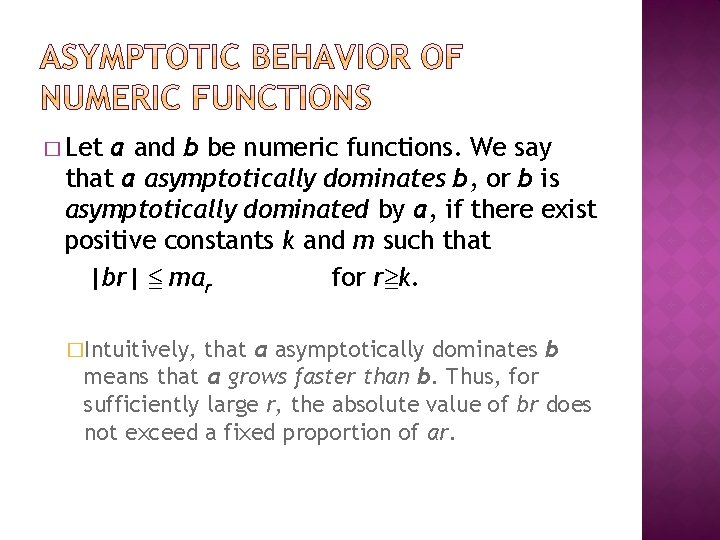� Let a and b be numeric functions. We say that a asymptotically dominates b, or b is asymptotically dominated by a, if there exist positive constants k and m such that |br| ≦ mar for r≧k. �Intuitively, that a asymptotically dominates b means that a grows faster than b. Thus, for sufficiently large r, the absolute value of br does not exceed a fixed proportion of ar.� asymptotically 1. 2. 3. 4. dominance For any numeric function a, |a| asymptotically dominates a. If b is asymptotically dominated by a, then for any constant α, αb is also asymptotically dominated by a. If b is asymptotically dominated by a, then for any integer i, Sib is asymptotically dominated by Sia. If b and c are asymptotically dominated by a, then for any constant α and β, αb+βc is also asymptotically dominated by a.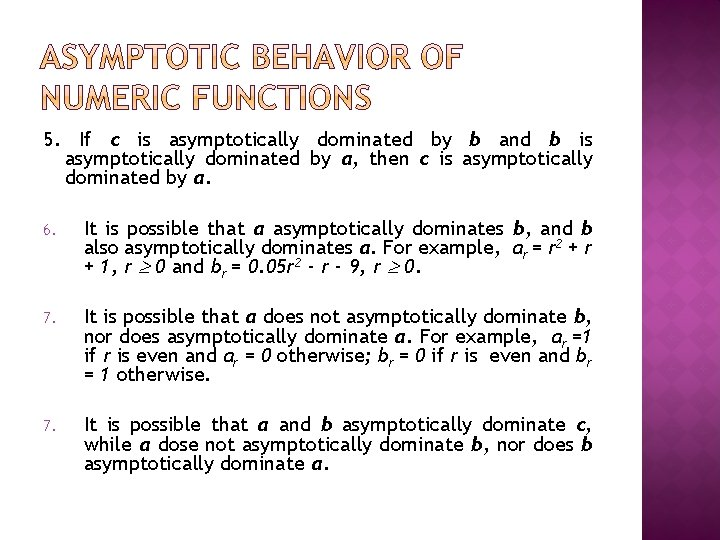5. If c is asymptotically dominated by b and b is asymptotically dominated by a, then c is asymptotically dominated by a. 6. It is possible that a asymptotically dominates b, and b also asymptotically dominates a. For example, ar = r 2 + r + 1, r 0 and br = 0. 05 r 2 - r - 9, r 0. 7. It is possible that a does not asymptotically dominate b, nor does asymptotically dominate a. For example, ar =1 if r is even and ar = 0 otherwise; br = 0 if r is even and br = 1 otherwise. 7. It is possible that a and b asymptotically dominate c, while a dose not asymptotically dominate b, nor does b asymptotically dominate a.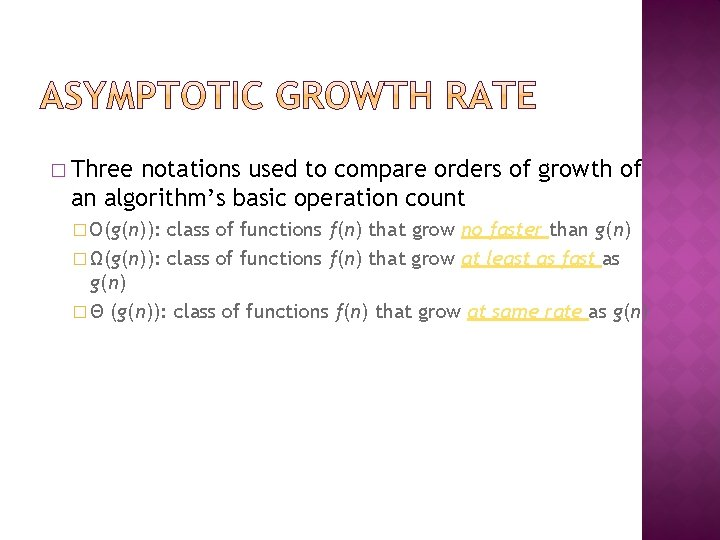� Three notations used to compare orders of growth of an algorithm’s basic operation count � O(g(n)): class of functions f(n) that grow no faster than g(n) � Ω(g(n)): class of functions f(n) that grow at least as fast as g(n) � Θ (g(n)): class of functions f(n) that grow at same rate as g(n)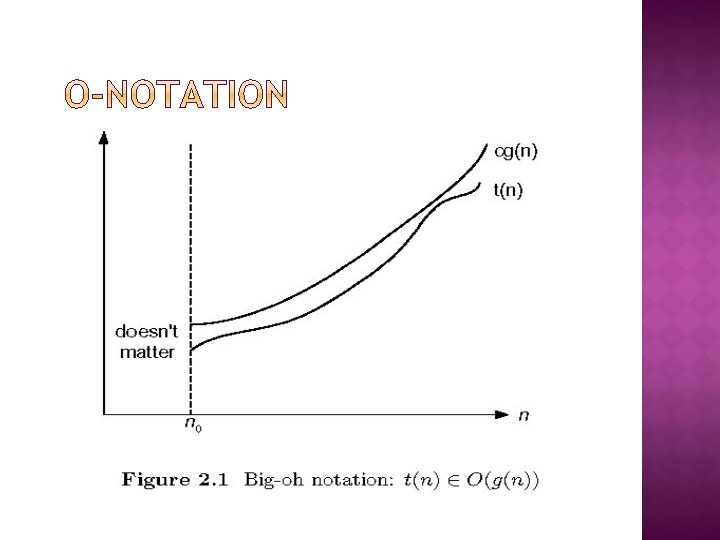� Formal definition function t(n) is said to be in O(g(n)), denoted t(n) O(g(n)), if t(n) is bounded above by some constant multiple of g(n) for all large n, i. e. , if there exist some positive constant c and some nonnegative integer n 0 such that t(n) cg(n) for all n n 0 �A � Example: � 10 n 2 O(n 2) � 10 n 2 + 2 n O(n 2) � 100 n + 5 O(n 2) � 5 n+20 O(n)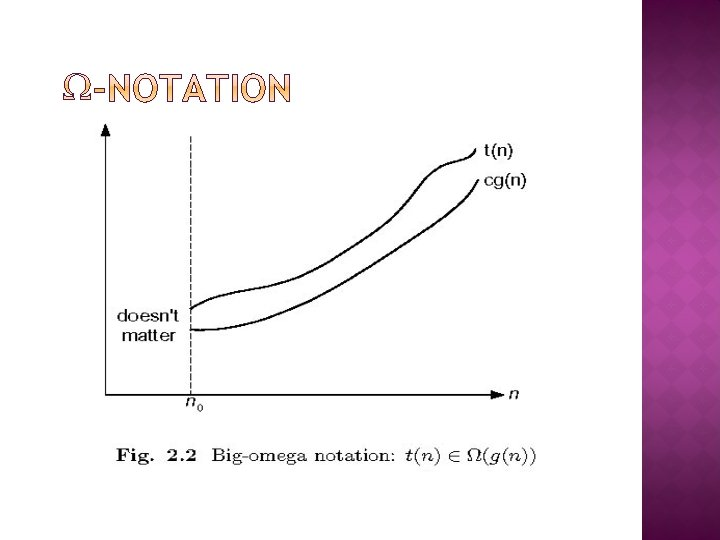� Formal definition function t(n) is said to be in (g(n)), denoted t(n) (g(n)), if t(n) is bounded below by some constant multiple of g(n) for all large n, i. e. , if there exist some positive constant c and some nonnegative integer n 0 such that t(n) cg(n) for all n n 0 �A � Exercises: prove the following using the above definition � 10 n 2 (n 2) � 10 n 2 + 2 n (n 2) � 10 n 3 (n 2)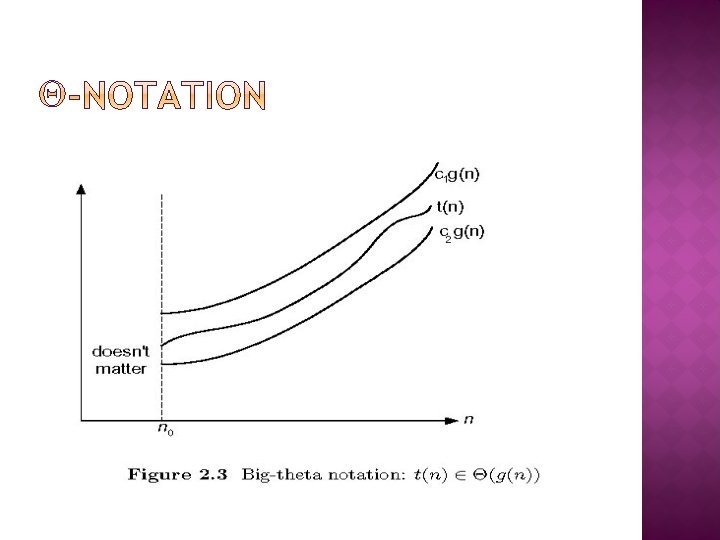� Formal definition function t(n) is said to be in (g(n)), denoted t(n) (g(n)), if t(n) is bounded both above and below by some positive constant multiples of g(n) for all large n, i. e. , if there exist some positive constant c 1 and c 2 and some nonnegative integer n 0 such that c 2 g(n) t(n) c 1 g(n) for all n n 0 �A � Exercises: prove the following using the above definition � 10 n 2 (n 2) � 10 n 2 + 2 n (n 2) � (1/2)n(n-1) (n 2)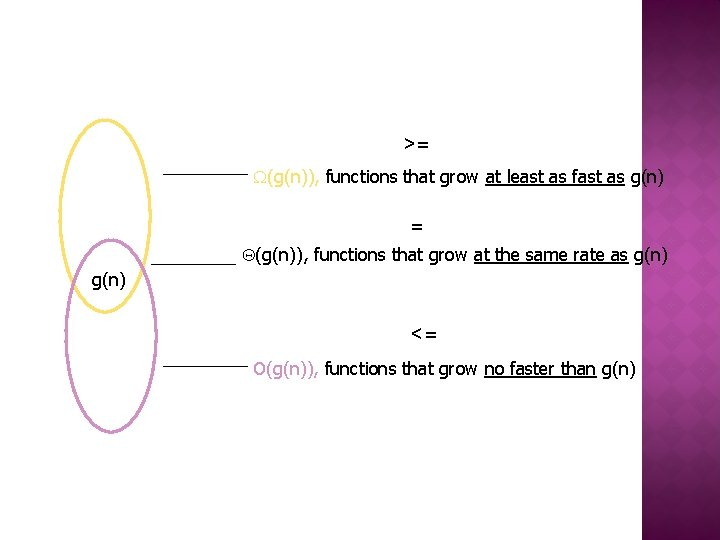>= (g(n)), functions that grow at least as fast as g(n) = (g(n)), functions that grow at the same rate as g(n) <= O(g(n)), functions that grow no faster than g(n)1. f(n) O(f(n)) 2. f(n) O(g(n)) iff g(n) (f(n)) 3. If f (n) O(g (n)) and g(n) O(h(n)) , then f(n) O(h(n)) Note similarity with a ≤ b 4. If f 1(n) O(g 1(n)) and f 2(n) O(g 2(n)) , then f 1(n) + f 2(n) O(max{g 1(n), g 2(n)}) The analogous assertions are true for the -notation and -notation.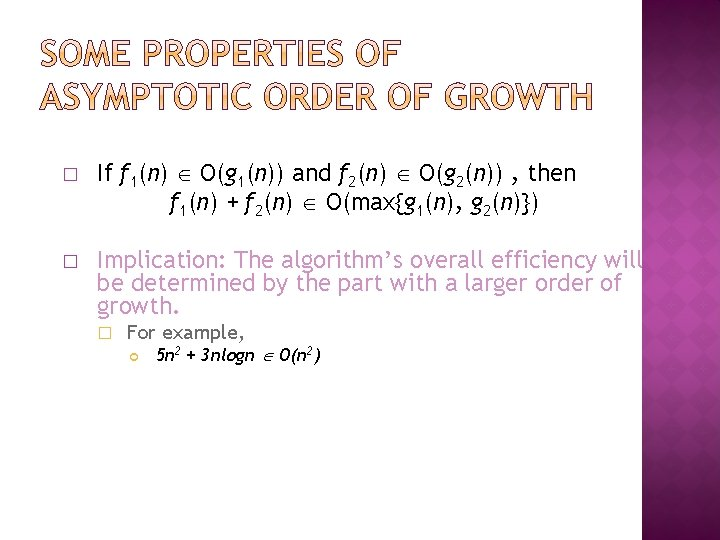� If f 1(n) O(g 1(n)) and f 2(n) O(g 2(n)) , then f 1(n) + f 2(n) O(max{g 1(n), g 2(n)}) � Implication: The algorithm’s overall efficiency will be determined by the part with a larger order of growth. � For example, 5 n 2 + 3 nlogn O(n 2)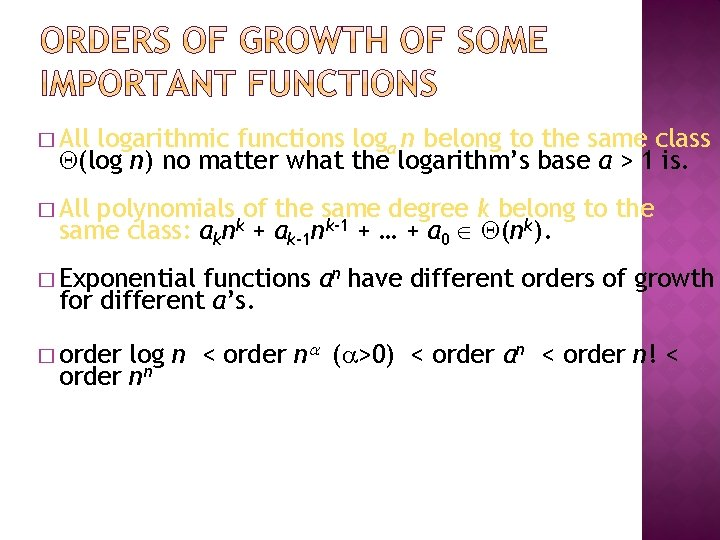� All logarithmic functions loga n belong to the same class (log n) no matter what the logarithm’s base a > 1 is. � All polynomials of the same degree k belong to the same class: aknk + ak-1 nk-1 + … + a 0 (nk). � Exponential functions an have different orders of growth for different a’s. log n < order n ( >0) < order an < order n! < order nn � orderfast slow 1 constant log n logarithmic n linear n log n n 2 quadratic n 3 cubic 2 n exponential n! factorial The time efficiencies of a large number of algorithms fall into only a few classes. High time efficiency low time efficiency� For a given discrete numeric function a, let O(a) denote the set of all numeric functions that are asymptotically dominated by a. O(a) is read “order a” or “big-oh of a”. � asymptotically domination again 1. For any numeric function a, a is O(a). 2. If b is O(a), then for any constant α, αb is also O(a). 3. If b is O(a), then for any integer i, Sib is O(Sia). 4. If both b and c are O(a), then for any constant α and β, αb+βc is also O(a).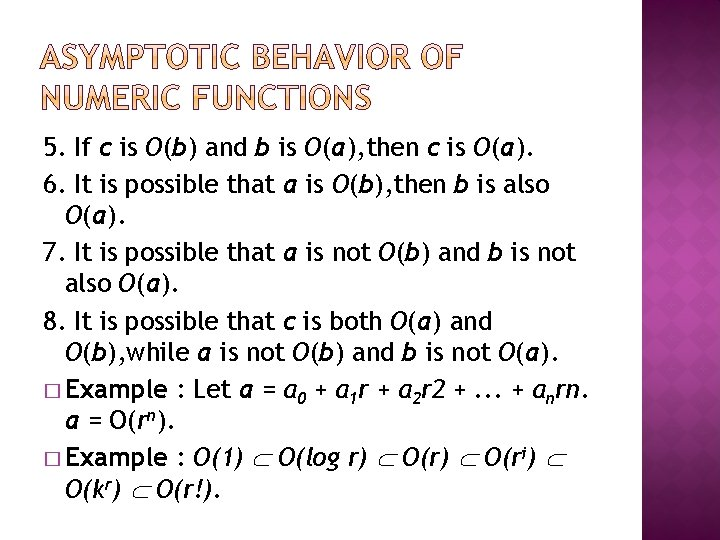5. If c is O(b) and b is O(a), then c is O(a). 6. It is possible that a is O(b), then b is also O(a). 7. It is possible that a is not O(b) and b is not also O(a). 8. It is possible that c is both O(a) and O(b), while a is not O(b) and b is not O(a). � Example : Let a = a 0 + a 1 r + a 2 r 2 +. . . + anrn. a = O(rn). � Example : O(1) O(log r) O(ri) O(kr) O(r!).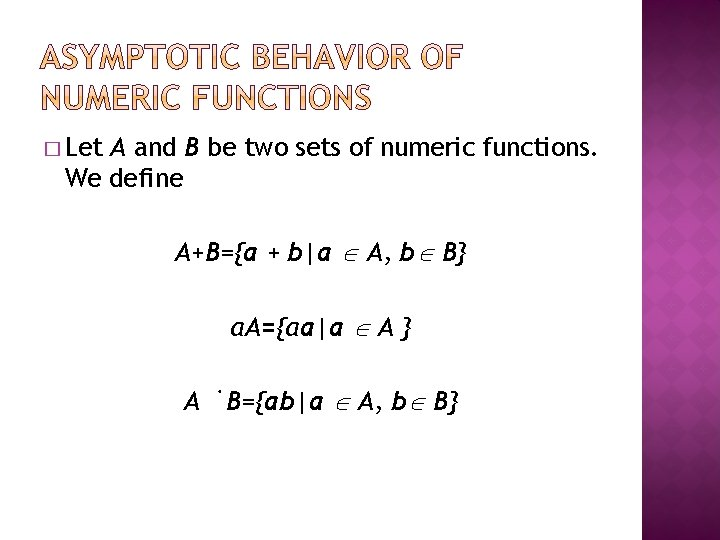� Let A and B be two sets of numeric functions. We define A+B={a + b|a A, b B} αA={αa|a A } A˙B={ab|a A, b B}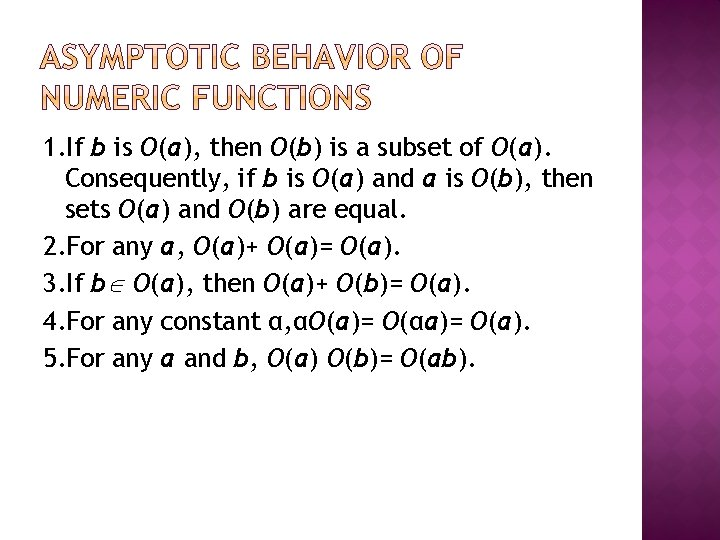1. If b is O(a), then O(b) is a subset of O(a). Consequently, if b is O(a) and a is O(b), then sets O(a) and O(b) are equal. 2. For any a, O(a)+ O(a)= O(a). 3. If b O(a), then O(a)+ O(b)= O(a). 4. For any constant α, αO(a)= O(αa)= O(a). 5. For any a and b, O(a) O(b)= O(ab).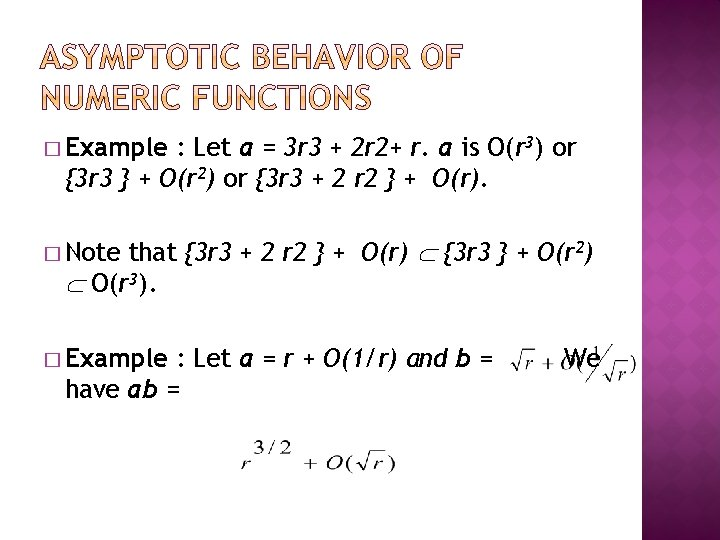� Example : Let a = 3 r 3 + 2 r 2+ r. a is O(r 3) or {3 r 3 } + O(r 2) or {3 r 3 + 2 r 2 } + O(r). that {3 r 3 + 2 r 2 } + O(r) {3 r 3 } + O(r 2) O(r 3). � Note � Example : Let a = r + O(1/r) and b = have ab = We� For a given numeric function a, Let Ω(a) denote the set of all numeric functions b such that there exist positive constants k and m with |br| ≧ mar for r ≧ k. � In other words, if b is in Ω(a), then b grows at least as fast as a.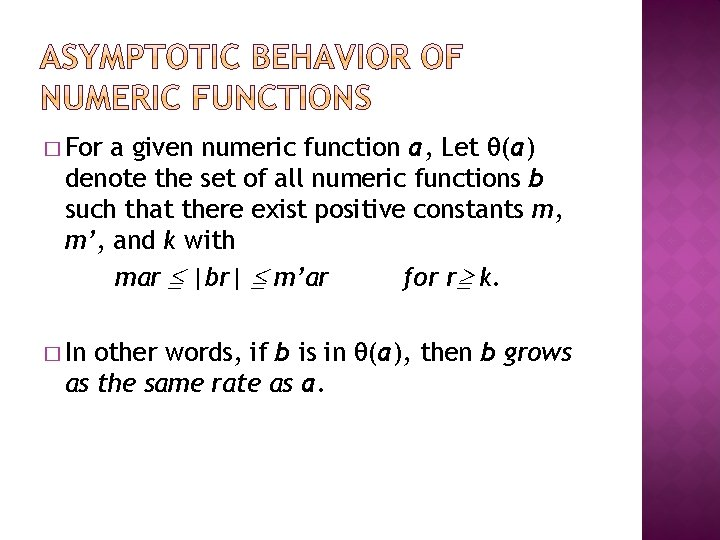� For a given numeric function a, Let θ(a) denote the set of all numeric functions b such that there exist positive constants m, m’, and k with mar ≦ |br| ≦ m’ar for r≧ k. � In other words, if b is in θ(a), then b grows as the same rate as a.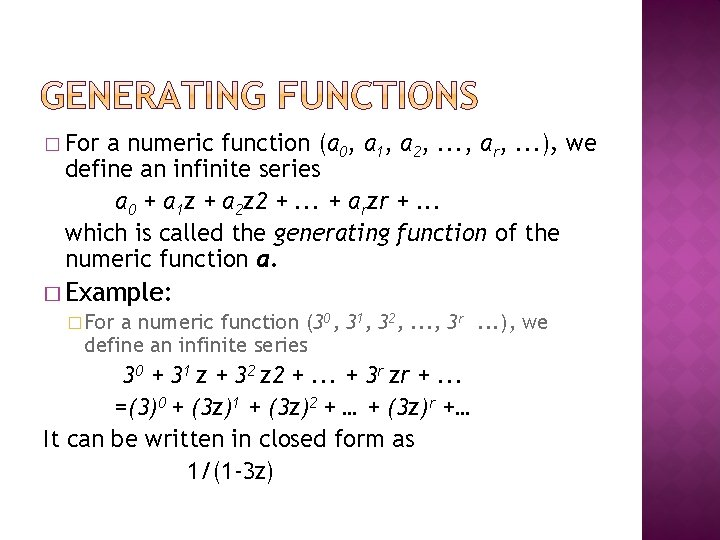� For a numeric function (a 0, a 1, a 2, . . . , ar, . . . ), we define an infinite series a 0 + a 1 z + a 2 z 2 +. . . + arzr +. . . which is called the generating function of the numeric function a. � Example: � For a numeric function (30, 31, 32, . . . , 3 r. . . ), we define an infinite series 30 + 31 z + 32 z 2 +. . . + 3 r zr +. . . =(3)0 + (3 z)1 + (3 z)2 + … + (3 z)r +… It can be written in closed form as 1/(1 -3 z)� Let a, b, and c be a numeric functions and A(z), B(z), and C(z) be its generating functions, respectively. 1. b = α a, B(z) = αa 0 + αa 1 z + αa 2 z 2 + … + αarzr + … = αA(z)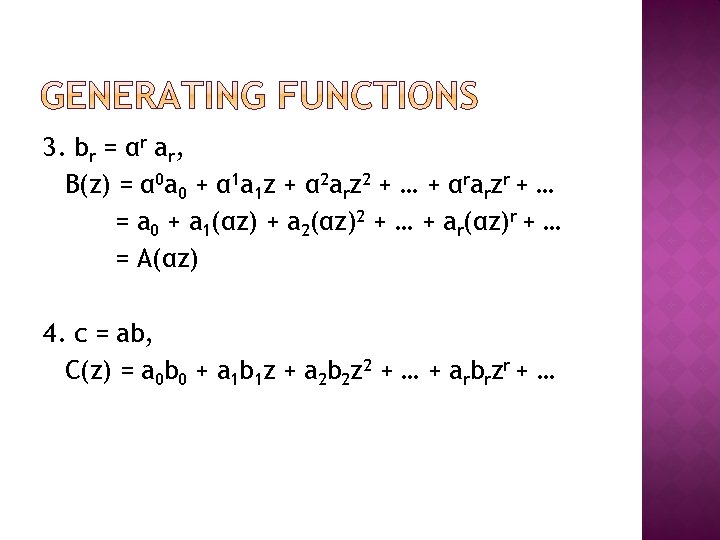3. br = αr ar, B(z) = α 0 a 0 + α 1 a 1 z + α 2 arz 2 + … + αrarzr + … = a 0 + a 1(αz) + a 2(αz)2 + … + ar(αz)r + … = A(αz) 4. c = ab, C(z) = a 0 b 0 + a 1 b 1 z + a 2 b 2 z 2 + … + arbrzr + …5. b = Sia, i ≥ 0. B(z) = 0 + 0 z + … + 0 zi-1 + a 0 zi + a 1 zi+1 + a 2 zi+2 + … + arzi+r + … = zi. A(z) 6. b = S-ia, i ≤ 0. B(z) = ai + ai+1 z + ai+2 z 2 + … + ai+rzr + … = z-i(A(z) - a 0 - a 1 z - a 2 z 2 - … - ai-1 zi-1)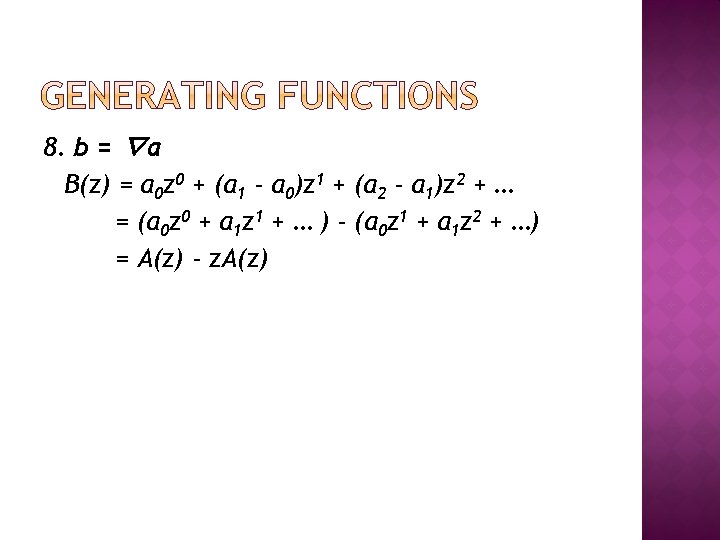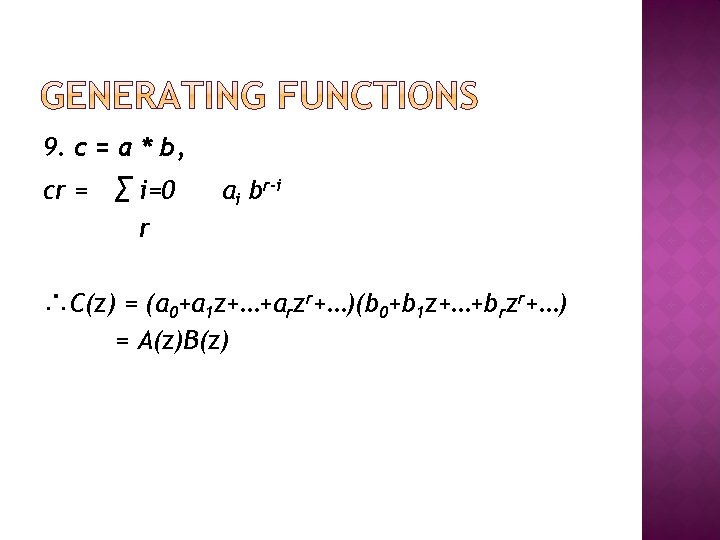9. c = a * b, cr = ∑ i=0 ai br-i r ∴C(z) = (a 0+a 1 z+…+arzr+…)(b 0+b 1 z+…+brzr+…) = A(z)B(z)